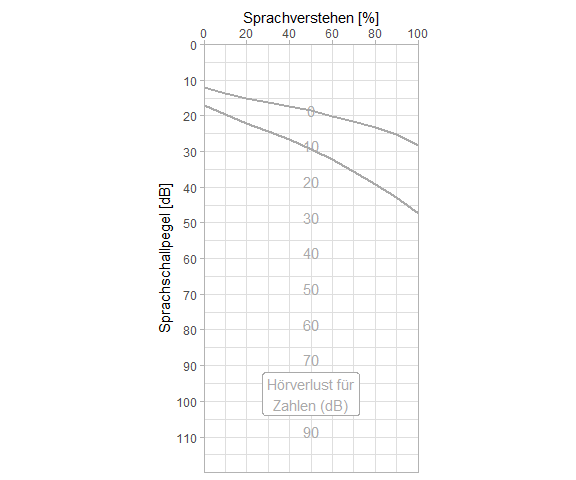# Freiburger Sprachtest

#### 2021-06-02

The Freiburger Sprachtest is arguably the most important speech audiometry test in German speaking countries. Just like pure tone audiometry it comes with an established look that can be resembled using the audiometry package in R.

Assuming some sample data

expl <- data.frame(test = gl(2,4, labels = c("Zahlen", "Einsilber")),
spl = c(20, 25, 30, 35, 35, 50, 65, 80),
int = c(30, 60, 85, 95, 40, 95, 100, 95))

a speech audiogram could be drawn like this:

library(ggplot2)
library(audiometry)
gg_freiburg(expl) +
geom_point(aes(x=spl, y=int, group=test), color="red") +
geom_line(aes(x=spl, y=int, group=test), color="red")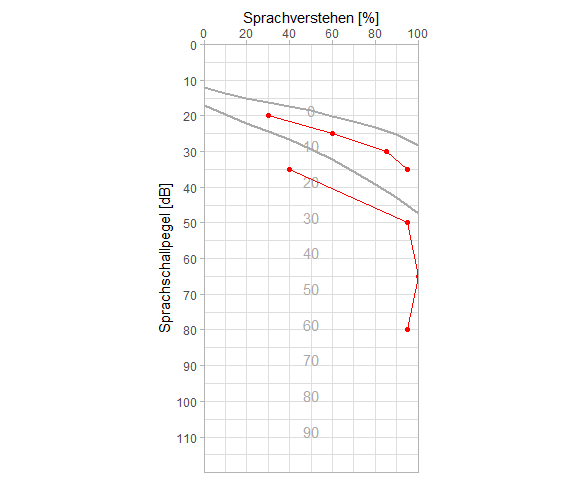There are some rules on how to draw this but also considerable variance. Some draw it horizontally, some vertically. There is DIN 45626-1:1995-08 which prints the diagramm horizontally and with the x axis label “Sprachschallpegel (Zeitbewertung I): Referenz: 20 uPa” and there is Muster 15: Ohrenärztliche Verordnung einer Hörhilfe which draws it vertically with x axis label “Sprachschallpegel in dB” and lots of other variation. This package does not aim to be everything for everyone, because there is ggplot2 which is excellent at that. However, there are some variations you can do with this package. Some examples are depicted along with the corresponding code.

Horizontal plots are the latest addition to the package (thus also the least tested):

data.frame(loud1 = c(10, 20, 30, 40), perc1 = c(0, 25, 75, 100),
loud2 = c(20, 35, 50, 65), perc2 = c(0,10,65,100)) |>
gg_freiburg(horizontal = TRUE) +
geom_point(aes(x = loud1, y = perc1)) +
geom_line(aes(x = loud1, y = perc1)) +
geom_point(aes(x = loud2, y = perc2)) +
geom_line(aes(x = loud2, y = perc2))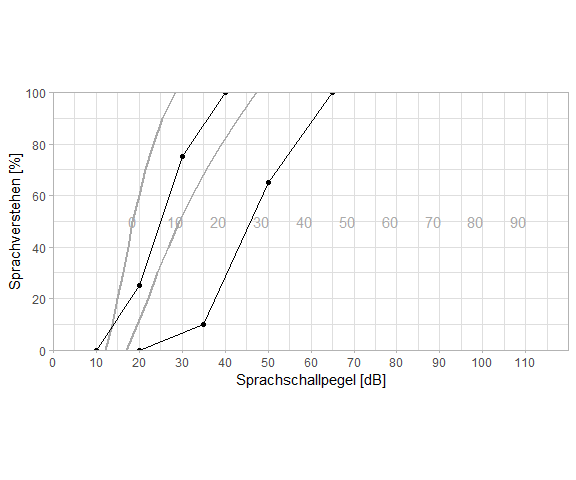## Aspect ratio

DIN45626-1:1995-08 clearly states a fixed aspect ratio: “Einer Änderung des Sprachschallpegels um 10 dB auf der Abszisse entspricht eine Änderung des Sprachverstehens von 20% auf der Ordinate”. This is widely neglected, Muster 15: Ohrenärztliche Verordnung einer Hörhilfe (Stand:10.2014) for one does not follow that rule. Apparently, ggplot2 sometimes behaves strangely with coord_fixed command in gg_freiburg. It is recommended to set an aspect ratio via the theme. If you wanted a square plot, you could achieved it like this:

gg_freiburg() +
theme(aspect.ratio = 1)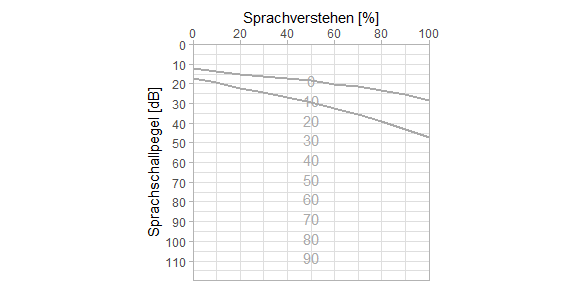## Change the axes

gg_freiburg(xlab = "How loud it was", ylab = "How much was understood",
x_ticks_at = seq(5, 120, 15), y_ticks_at = c(0, 25, 50, 75, 100),
plot_discr_loss_scale = FALSE)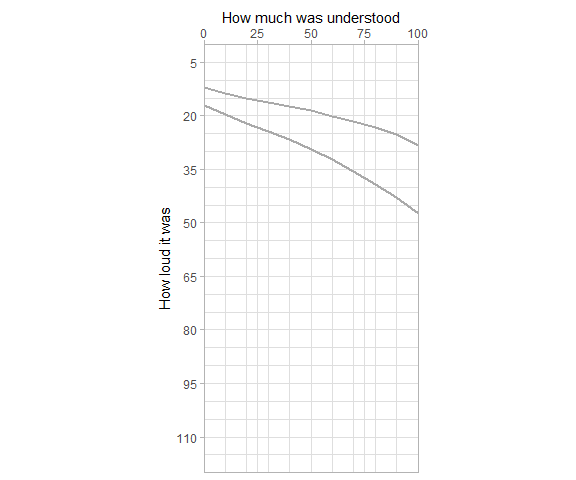Note how loudness remains the x axis and percentage remains the y axis in case of a horizontal plot:

gg_freiburg(xlab = "How loud it was", ylab = "How much was understood",
x_ticks_at = seq(5, 120, 15), y_ticks_at = c(0, 25, 50, 75, 100),
plot_discr_loss_scale = FALSE, horizontal = TRUE)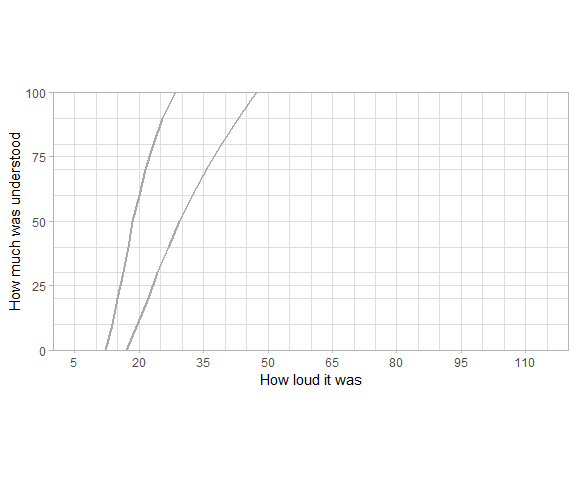## Highlight or hide normal values

Should your data contain only “Zahlwörter” or only “Einsilber” values, you might choose to highlight the normal curves or let them dissappear altogether. There is an example for playing with color and line width in the function documentation, available via

library(audiometry)
example(gg_freiburg)

## An extra relative scale for discrimination loss

A pecularity of this plot is an additional scale in the middle of the plot, highlighted here in color:

gg_freiburg(plot_discr_loss_scale = TRUE, plot_discr_loss_scale_color = "red")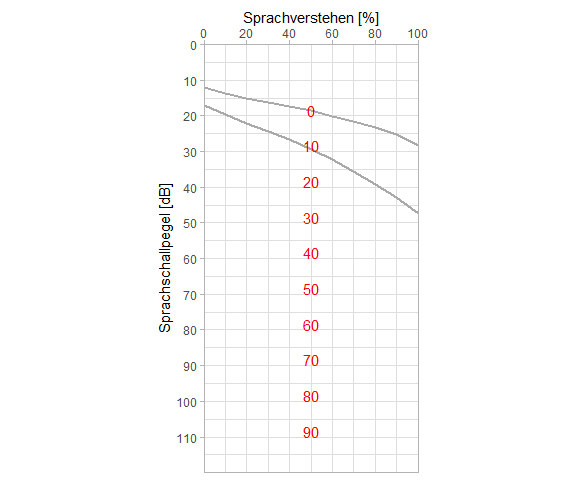Sometimes a label is added to that, in which case there is not enough room for all the numbers:

gg_freiburg(plot_discr_loss_scale_values = seq(0, 60, 10)) +
geom_text(aes(x=100, y=45, label = "H\u00f6rverlust (dB)"), color="darkgrey", angle = 90)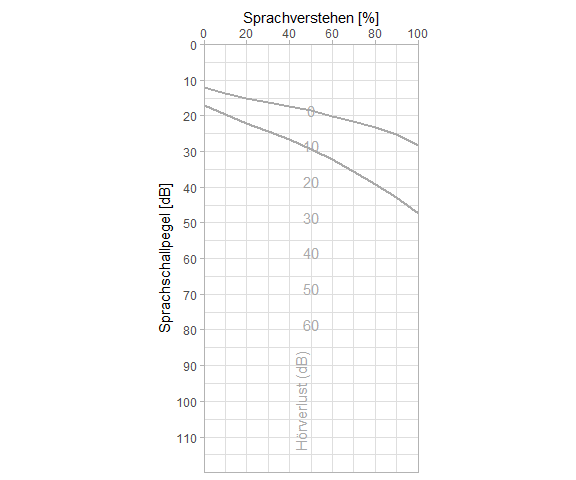or maybe

gg_freiburg() +
geom_label(aes(x=98, y=50, label = "H\u00f6rverlust f\u00fcr\nZahlen (dB)"),
color="darkgrey")